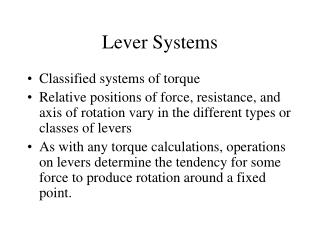DownloadDownload PresentationLever Systems

# Lever Systems

Download Presentation## Lever Systems

- - - - - - - - - - - - - - - - - - - - - - - - - - - E N D - - - - - - - - - - - - - - - - - - - - - - - - - - -
##### Presentation Transcript

1. Lever Systems Classified systems of torque Relative positions of force, resistance, and axis of rotation vary in the different types or classes of levers As with any torque calculations, operations on levers determine the tendency for some force to produce rotation around a fixed point.

2. Components of a Lever System • Fulcrum – The center or axis of rotation of the system. • Moment Arm – The distance from any force or weight that produces torque to the fulcrum. • Force Arm – The distance from an applied force to the fulcrum. (The moment arm of the force.) • Resistance Arm – The distance from the resistance to the fulcrum. (The moment arm of the resistance.)

3. Classes of Levers • First Class – The applied force and the resistance are on opposite sides of the fulcrum. • Second Class – The resistance is between the applied force and the fulcrum. • Third Class – The applied force is between the resistance and the fulcrum.

4. applied force resistance force arm resistance arm fulcrum First Class Lever

5. force arm resistance arm resistance applied force fulcrum Second Class Lever

6. resistance arm force arm resistance applied force fulcrum Third Class Lever

7. Torque Produced in Lever Systems • Two Torques • Torque produced by the applied force • Torque produced by the resistance • The direction in which a lever system moves is dependent on the relative lengths of the force and resistance arms as well as the magnitudes of force and resistance.

8. Mechanical Advantage This is the effectiveness of a lever at moving a resistance. It is a calculated value: Mechanical Advantage = Because of their different configurations, the mechanical advantage of a first class lever can favor the force or resistance depending on the placement of the fulcrum. A second class lever always favors the force arm. A third class lever always favors the resistance arm.

9. fulcrum applied force resistance force arm resistance arm The fulcrum in a first class lever system can often vary in position to favor the force arm or the resistance arm.

10. force arm resistance arm resistance applied force fulcrum In a second class lever system, the mechanical advantage favors the force arm. (The force arm will always be longer.)

11. resistance arm force arm resistance applied force fulcrum The mechanical advantage of a third class lever system favors the resistance arm. (The resistance arm is always longer.)

12. Resistance and Force Arms • Resistance Arm: Abbreviated DRA • Force Arm: Abbreviated DFA

13. Levers In The Musculo-Skeletal System • Most are third class levers • This system produces a disadvantage for force but an advantage for speed of movement

14. FM FRO DFA R DRA Levers In The Musculo-Skeletal System Most of the musculo-skeletal system consists of third class levers. That is, the resistance arm is longer than the force arm.

15. B A Levers In The Musculo-Skeletal System The musculo-skeletal lever systems generally favor speed over strength. Although the mechanical advantage favors the resistance arm, in the time that the muscle insertion moves a given distance (red arrow), the resistance moves a much greater distance (blue arrow).

16. B A Levers In The Musculo-Skeletal System In other words, the end of a limb is moving at a greater velocity than the attachments of the muscles that produce that movement.

17. Strength vs. Speed in Skeletal Muscle • mechanical advantage = (DFA)/(DRA) If DFA < DRA the mechanical advantage is < 1 Example: A muscle inserts 3 cm from a joint axis (DFA = 3 cm) The distance to the weight that the muscle is resisting is 30 cm (DRA = 30 cm) mechanical advantage = 3 cm / 30 cm = .1 This means that, when FM and R are both perpendicular to the limb, FM must be 10 times greater than R to move the resistance.

18. q Strength vs. Speed in Skeletal Muscle • In a muscle contraction on a limb like the arm or leg, the resistance moves through the same angular displacement as the muscle insertion.

19. Strength vs. Speed in Skeletal Muscle • In moving through a given angular displacement (q), the velocity of the resistance is equal to the angular velocity in rad/sec times DRA • The velocity of the muscle insertion is equal to the angular velocity in rad/sec times DFA q

20. FM FRO DFA R DRA Strength vs. Speed in Skeletal Muscle

21. Strength vs. Speed in Skeletal Muscle • The velocity of the resistance: vR = (w rad/sec)(DRA) • The velocity of the muscle insertion: vM = (w rad/sec)(DFA) • The velocity of the resistance compared to the muscle insertion = vR/vM = (w rad/sec)(DRA)/ (w rad/sec)(DFA) = DRA/DFA

22. Strength vs. Speed in Skeletal Muscle • If DFA = 3 cm and DRA = 30 cm, The relative speed of the resistance to the muscle insertion = DRA/DFA = (30 cm)/(3 cm) = 10 This means that the resistance is moving at 10 times the velocity of the muscle insertion.

23. Levers in the Musculo-Skeletal System • Not all levers in the musculo-skeletal system are third class. When performing toe rises the ankle becomes a second class lever system. R FM DRA DFA fulcrum